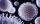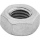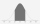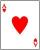Probability of malaria

A survey carried out at a certain hospital indicates that the probability that a patient tested positive for malaria is 0.6. What is the probability that two patients selected at random
(i) one is negative while the other tested positive?
(i) both patients tested positive.

Result

p1 =  0.48
p2 =  0.36

Solution:Leave us a comment of this math problem and its solution (i.e. if it is still somewhat unclear...):Be the first to comment!To solve this verbal math problem are needed these knowledge from mathematics:

Looking for a statistical calculator? Would you like to compute count of combinations?

Next similar math problems:

1. One greenIn the container are 45 white and 15 balls. We randomly select 5 balls. What is the probability that it will be a maximum one green?
2. CardsThe player gets 8 cards of 32. What is the probability that it gets a) all 4 aces b) at least 1 ace
3. Genetic diseaseOne genetic disease was tested positive in both parents of one family. It has been known that any child in this family has a 25% risk of inheriting the disease. A family has 3 children. What is the probability of this family having one child who inherited.
4. TestThe teacher prepared a test with ten questions. The student has the option to choose one correct answer from the four (A, B, C, D). The student did not get a written exam at all. What is the probability that: a) He answers half correctly. b) He answers.
5. Component failThere is a 90 percent chance that a particular type of component will perform adequately under high temperature conditions. If the device involved has four such components, determine the probability that the device is inoperable because exactly one of the.
6. DiceWe throw 10 times a play dice, what is the probability that the six will fall exactly 4 times?
7. Family94 boys are born per 100 girls. Determine the probability that there are two boys in a randomly selected family with three children.
8. Internet anywhereIn school, 60% of pupils have access to the internet at home. A group of 8 students is chosen at random. Find the probability that a) exactly 5 have access to the internet. b) At least 6 students have access to the internet
9. A bookA book contains 524 pages. If it is known that a person will select any one page between the pages numbered 125 and 384, find the probability of choosing the page numbered 252 or 253.
10. BallsThe urn is 8 white and 6 black balls. We pull 4 randomly balls. What is the probability that among them will be two white?
11. A pizzaA pizza place offers 14 different toppings. How many different three topping pizzas can you order?
12. Hearts5 cards are chosen from a standard deck of 52 playing cards (13 hearts) with replacement. What is the probability of choosing 5 hearts in a row?
13. Theorem proveWe want to prove the sentence: If the natural number n is divisible by six, then n is divisible by three. From what assumption we started?
14. Fish tankA fish tank at a pet store has 8 zebra fish. In how many different ways can George choose 2 zebra fish to buy?
15. TrinityHow many different triads can be selected from the group 43 students?
16. Calculation of CNCalculate: ?
17. ChordsHow many 4-tones chords (chord = at the same time sounding different tones) is possible to play within 7 tones?